# Multi-Dielectric Coax

Click here to go to our page on dispersion (dispersion will exist in any multi-dielectric transmission line)

Thanks to contributor Alex R. from a major connector manufacturer, we have now have a spreadsheet that can calculate parameters for multi-dielectric coax, beyond just two dielectrics! If you want to download Alex's spreadsheet, go to our download area and look for a spreadsheet called Multi-dielectric_coax_Rev1.xls. Alex solved the equations for two, three, four and five different layers of dielectric. If you need more than five dielectric layers, you are on your own, but if you modify the spreadsheet, send it back and we might post your version too!

One of these days we will fix up the graphics on this page, but don't hold your breath. You can skip all the boring math and get your answer by using our dual-dielectric coax calculator!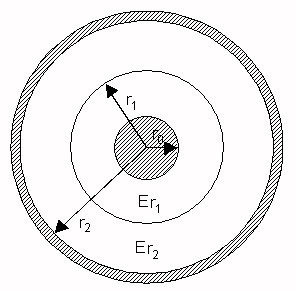When a coaxial cable is constructed using two concentric dielectrics as shown above, the wave propagation is no longer purely transverse-electromagnetic (TEM), as in the single-dielectric coax, but instead is quasi-TEM, like microstrip or CPW and can suffer from dispersion. But the "non-TEM-ness" is very slight indeed. In the most general terms the characteristic impedance and effective dielectric constant are very slight functions of frequency, and if this is important to you, you should analyze your structure using a 3D EM solver. However, the static Z0 and Keff terms can be derived easily, and they are useful over a fairly wide range of frequencies. The derivation of these terms is as follows...

Coax impedance is the square-root of the ratio of inductance/length divided by capacitance/length: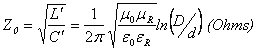Note that because the ln(D/d) term is a ratio, you can just as well use ln(R/r), and in either case the dimensional units don't matter.

The "trick" to doing the multi-dielectric calculation is to note that capacitance of multiple concentric dielectrics can be easily be calculated by adding their individual capacitances in series. The coax inductance calculation does not change no matter what dielectric you put between the center and outer conductors.

In the static (DC) case, all of the electric flux lines are perpendicular to the center conductor - dielectric 1, dielectric 1 - dielectric 2, and dielectric 2 - outer conductor interfaces, as shown: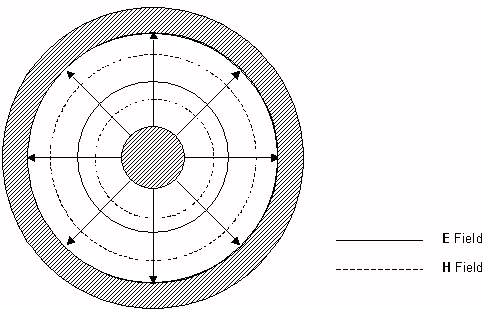To calculate the static capacitance between the inner and outer conductors, imagine a zero-thickness conducting cylinder placed inside the cable, at the interface between the two dielectrics. Since all of the electric field components are already perpendicular to this interface, this does not violate the boundary conditions of the initial problem. With this conducting cylinder in place, two capacitance values can now be calculated: 1) the capacitance (per meter length of cable) between the inner conductor and the cylinder; and 2) the capacitance between the cylinder and the outer conductor. The capacitance per meter of cable between the inner and outer conductors is then the series combination of the above two capacitance values.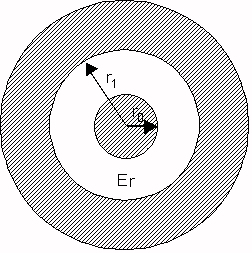The capacitance per length of a single-dielectric coaxial cable (dimensions shown above) is as follows: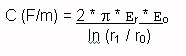Therefore, the capacitance per meter length between the inner conductor and the cylinder is: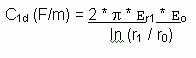The capacitance per meter length between the cylinder and the outer conductor is: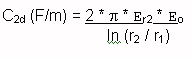The capacitance per meter length of the cable itself is the series combination of the above two capacitances: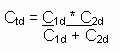Leaving out the algebraic steps, this comes out to: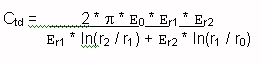The effective dielectric constant of the structure is the ratio of the capacitance of the structure to the capacitance if the dielectrics were replaced with a vacuum (or air), i.e.: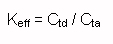where: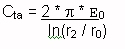This gives the following expression for the effective dielectric constant: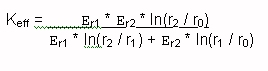With a bit of additional effort, the characteristic impedance can also be found. For this we need the inductance per meter length, which is unaffected by the dielectric(s) in the structure. The expression for the inductance is: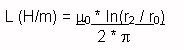The characteristic impedance of any transmission line is equal to the square root of the ratio of the inductance per length to the capacitance per length. Again leaving out the algebraic steps,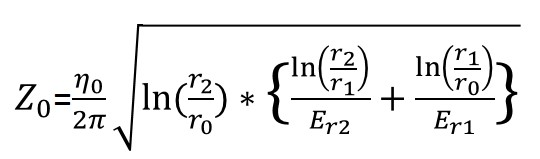### Dispersion in dual-dielectric coax

I validated these expressions using HFSS for the following geometry: r0 = 0.040", r1 = 0.100", r2 = 0.140", Er1 = 2.0, and Er2 = 5.0. For those dimensions, the above expressions yield Z0 = 48.65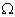, and Keff = 2.384. HFSS results for the same configuration are as follows:

Frequency, GHz Z0, Ohms (P-V) Keff
1.0 48.62 2.384
2.0 48.63 2.385
3.0 48.64 2.386
4.0 48.65 2.387
5.0 48.67 2.389
6.0 48.69 2.391
7.0 48.71 2.393
8.0 48.73 2.396
9.0 48.76 2.399
10.0 48.79 2.403
11.0 48.82 2.407
12.0 48.86 2.412
13.0 48.89 2.417
14.0 48.92 2.423
15.0 48.96 2.429

As seen from the numbers, the expressions for the static Z0 and Keff are valid at low frequencies, and for the dimensions used in this example, the dispersion is mild below 15 GHz. Smaller dimensions and closer values of Er1 and Er2 would reduce the dispersion further.

The derivation of the expressions for Z0 and Keff in the case in which the division between Er1 and Er2 is angular, rather than radial, is probably more complicated.

Good luck!

Author : Unknown Editor